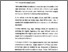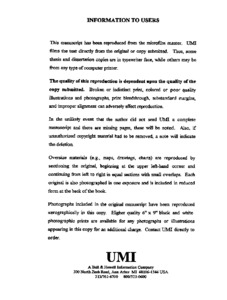Title:

# Spectral approximation by the polar transformation

Zhou, Wei Hua (1997) Spectral approximation by the polar transformation. Masters thesis, Concordia University.Preview
Text (application/pdf)
MQ25988.pdf
1MB

## Abstract

Central potentials V(r) are considered which admit the polar representation $V(r)=g(h(r)),$ where $h(r)={\rm sgn}(q)r\sp{q},$ q is fixed, and g is the polar transformation function. This representation allows the Schrodinger eigenvalues generated by V to be approximated in terms of those generated by the polar potential h(r). In many cases the optimal values $\{q\sb1,q\sb2\}$ of the power q can be chosen so that the corresponding polar functions $\{g\sb1,g\sb2\}$ have definite and opposite convexity. For such cases the spectral approximations provide both upper and lower bounds for the entire discrete spectrum. The example of the central potential $V(r)=ar\sp2+br\sp2/(1+cr\sp2)$ in $R\sp3$ is studied in detail: optimal bounds are determined for a wide range of the potential parameters. The method is applicable, essentially unchanged, for problems in any number of spatial dimensions.

Divisions: Concordia University > Faculty of Arts and Science > Mathematics and Statistics Thesis (Masters) Zhou, Wei Hua vi, 56 leaves ; 29 cm. Concordia University M.Sc. Mathematics 1997 Hall, Richard L. QC 20.7 S64Z48 1997 263 Concordia University Library 27 Aug 2009 17:10 13 Jul 2020 19:46 https://concordiauniversity.on.worldcat....
All items in Spectrum are protected by copyright, with all rights reserved. The use of items is governed by Spectrum's terms of access.

Repository Staff Only: item control pageResearch related to the current document (at the CORE website)
Back to top